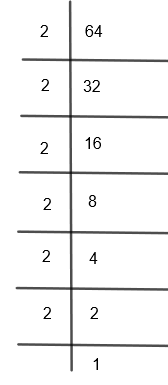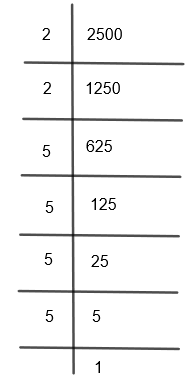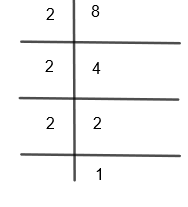QUESTION

# Simplify the following expression:$3\sqrt{2}+\sqrt{64}+\sqrt{2500}+\sqrt{8}$(a) $11\sqrt{2}$(b) $2\sqrt{11}$(c) $11\sqrt{2}$(d) $2\sqrt{11}$

Hint: First of all, rewrite the given expression using $\sqrt[n]{a}={{a}^{\dfrac{1}{n}}}$. Now prime factorize each term and then use ${{\left( {{a}^{m}}.{{b}^{n}} \right)}^{p}}={{a}^{mp}}.{{b}^{np}}$. Simplify the expression and take out the common terms to get the final answer.

Here, we have to simplify and find the final value of the expression:
$3\sqrt{2}+\sqrt{64}+\sqrt{2500}+\sqrt{8}$
Let us consider the expression given in the question:
$E=3\sqrt{2}+\sqrt{64}+\sqrt{2500}+\sqrt{8}$
We know that $\sqrt[n]{a}={{\left( a \right)}^{\dfrac{1}{n}}}$. By using this, we can write the above expression as,
$E=3{{\left( 2 \right)}^{\dfrac{1}{2}}}+{{\left( 64 \right)}^{\dfrac{1}{4}}}+{{\left( 2500 \right)}^{\dfrac{1}{4}}}+{{\left( 8 \right)}^{\dfrac{1}{6}}}.....\left( i \right)$
Now, we will do the prime factorization of each term to further simplify them. First of all, let us do the prime factorization of 64. We get,So, we can write,
$64=2\times 2\times 2\times 2\times 2\times 2={{2}^{6}}....\left( ii \right)$
Now, let us do the prime factorization of 2500. We get,So, we can write
$2500=2\times 2\times 5\times 5\times 5\times 5={{\left( 2 \right)}^{2}}.{{\left( 5 \right)}^{4}}....\left( iii \right)$
Now, let us do the prime factorization of 8, we get,So, we can write
$8=2\times 2\times 2={{\left( 2 \right)}^{3}}....\left( iv \right)$
Now by substituting the values of 64, 2500 and 8 from equation (ii), (iii) and (iv) in equation (i), we get,
$E=3{{\left( 2 \right)}^{\dfrac{1}{2}}}+{{\left( {{2}^{6}} \right)}^{\dfrac{1}{4}}}+{{\left( {{2}^{2}}{{.5}^{4}} \right)}^{\dfrac{1}{4}}}+{{\left( {{2}^{3}} \right)}^{\dfrac{1}{6}}}$
We know that ${{\left( {{a}^{m}}.{{b}^{n}} \right)}^{p}}={{a}^{mp}}.{{b}^{np}}$. By using this in the above equation, we get,
$E=3{{\left( 2 \right)}^{\dfrac{1}{2}}}+{{\left( 2 \right)}^{\dfrac{6}{4}}}+{{\left( 2 \right)}^{\dfrac{2}{4}}}.{{\left( 5 \right)}^{\dfrac{4}{4}}}+{{\left( 2 \right)}^{\dfrac{3}{6}}}$
$E=3{{\left( 2 \right)}^{\dfrac{1}{2}}}+{{\left( 2 \right)}^{\dfrac{3}{2}}}+{{\left( 2 \right)}^{\dfrac{1}{2}}}.5+{{\left( 2 \right)}^{\dfrac{1}{2}}}$
$\Rightarrow E=3{{\left( 2 \right)}^{\dfrac{1}{2}}}+{{\left( 2 \right)}^{1+\dfrac{1}{2}}}+{{\left( 2 \right)}^{\dfrac{1}{2}}}.5+{{\left( 2 \right)}^{\dfrac{1}{2}}}$
We know that ${{a}^{m+n}}={{a}^{m}}.{{a}^{n}}$. By using this in the above expression, we get,
$\Rightarrow E=3{{\left( 2 \right)}^{\dfrac{1}{2}}}+{{2}^{1}}.{{\left( 2 \right)}^{\dfrac{1}{2}}}+{{\left( 2 \right)}^{\dfrac{1}{2}}}.5+{{\left( 2 \right)}^{\dfrac{1}{2}}}$
By taking out ${{2}^{\dfrac{1}{2}}}$ common from the above expression, we get,
$E=\left( {{2}^{\dfrac{1}{2}}} \right)\left[ 3+2+5+1 \right]$
$E=\left( 11 \right){{2}^{\dfrac{1}{2}}}$
$\Rightarrow E=11\sqrt{2}$
Hence, option (a) is the right answer.

Note: In this question, many students make this mistake of writing the wrong powers of the numbers after prime factorization of them. So, this must be taken care of and better to recheck them each time. Also, some students misread $n\sqrt{m}$ as $\sqrt[n]{m}$ which is very different. Here, $n\sqrt{m}=n\times \sqrt{m}$ and $\sqrt[n]{m}={{\left( m \right)}^{\dfrac{1}{n}}}$. So, read the question properly and then solve the expression.